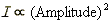The wave intensity is proportional to the square of the amplitude of the wave.

Note: The intensity of a wave is the wave's power-per-area passing through or impinging upon an imaginary or a real surface.

Note: "Proportional to" means "equal to a constant times". Thus the statement that A is proportional to B means that A = (a constant) times B. As a consequence of being proportional to the square of the amplitude, the intensity becomes four times its original value if the amplitude of the wave is doubled.